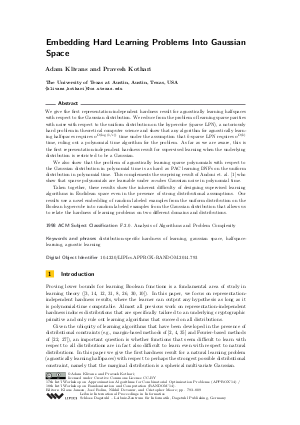Document# Embedding Hard Learning Problems Into Gaussian Space

### Authors Adam Klivans, Pravesh Kothari## File

LIPIcs.APPROX-RANDOM.2014.793.pdf
• Filesize: 482 kB
• 17 pages

## Cite As

Adam Klivans and Pravesh Kothari. Embedding Hard Learning Problems Into Gaussian Space. In Approximation, Randomization, and Combinatorial Optimization. Algorithms and Techniques (APPROX/RANDOM 2014). Leibniz International Proceedings in Informatics (LIPIcs), Volume 28, pp. 793-809, Schloss Dagstuhl - Leibniz-Zentrum für Informatik (2014)
https://doi.org/10.4230/LIPIcs.APPROX-RANDOM.2014.793

## Abstract

We give the first representation-independent hardness result for agnostically learning halfspaces with respect to the Gaussian distribution. We reduce from the problem of learning sparse parities with noise with respect to the uniform distribution on the hypercube (sparse LPN), a notoriously hard problem in theoretical computer science and show that any algorithm for agnostically learning halfspaces requires n^Omega(log(1/\epsilon)) time under the assumption that k-sparse LPN requires n^Omega(k) time, ruling out a polynomial time algorithm for the problem. As far as we are aware, this is the first representation-independent hardness result for supervised learning when the underlying distribution is restricted to be a Gaussian. We also show that the problem of agnostically learning sparse polynomials with respect to the Gaussian distribution in polynomial time is as hard as PAC learning DNFs on the uniform distribution in polynomial time. This complements the surprising result of Andoni et. al. 2013 who show that sparse polynomials are learnable under random Gaussian noise in polynomial time. Taken together, these results show the inherent difficulty of designing supervised learning algorithms in Euclidean space even in the presence of strong distributional assumptions. Our results use a novel embedding of random labeled examples from the uniform distribution on the Boolean hypercube into random labeled examples from the Gaussian distribution that allows us to relate the hardness of learning problems on two different domains and distributions.
##### Keywords
• distribution-specific hardness of learning
• gaussian space
• halfspace-learning
• agnostic learning

## Metrics

• Access Statistics
• Total Accesses (updated on a weekly basis)
0